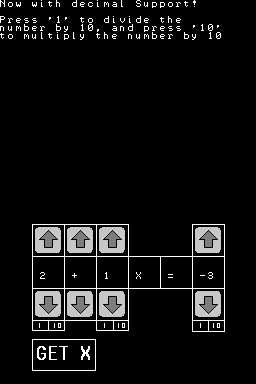# Maths Mate: Auto Algebra!

### From GameBrew

Maths Mate: Auto Algebra!General
Authorjpoopdog
Last Updated2011/09/10
TypeMath
Version0.2A
Website

Mathematical application which able to work out simple algebra equation.

## User guide

To operate the game, press the back button, otherwise user will be able to write up numbers in the 100's, rather than the 10's.

Once the variable reaches 4, user can no longer make input, until select an operator, which is represented by a number. Only addition is supported.

If you want a number that uses 10's digits, type as normal. If you wants 1's digits, press 0 first. The application will automatically switch to the next square after typed in 2 numbers.

The final square is for the answer of equation.

Pressing any non number buttons when you haven't typed 0 before a single digit number will result in it being repeated.

If you press A, at the bottom of the bottom screen a number will apear, this is the value of X.

An example, to try out, is 3 + 5x = 13.

In this, x = 2, because 3 + 5 x 2 is 13 (2x5 is 10), which is basic math.

In that equation, the value of x will come out as 2.

Lastly, remember this only works for equations that use + and positive numbers, despite the fact there are + - x / avaliable (due to the fact they are not practical).

## Controls

Up/Down - Increase or decrease the number in the box

A - Confirm

1 (stylus) - Divide by 10

10 (stylus) - Multiply the number in the box by 10, to make reaching large numbers quicker

Get X (stylus) - Change the x into the value of X

## Changelog

• Because the background interferes with the value of x where it is, x is now redrawn on the top screen.

2011/05/09 1 hour after updates

• Now values increase when the button is held.
• The value of x is redrawn on the top screen for easy readability.